• +91 9971497814
• info@interviewmaterial.com

# RD Chapter 4- Algebraic Identities Ex-VSAQS Interview Questions Answers

### Related Subjects

Question 1 :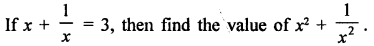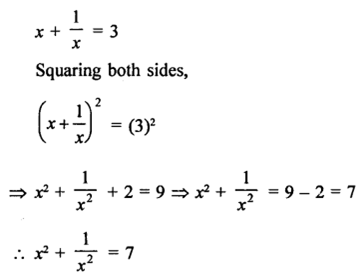Question 2 :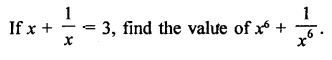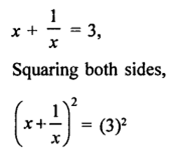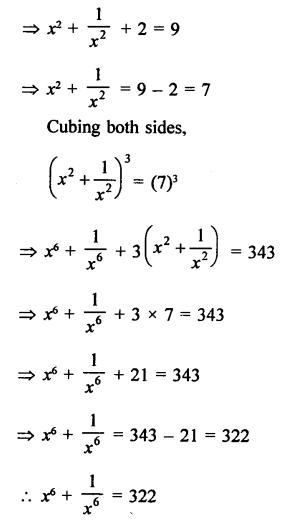Question 3 : If a + b = 7 and ab = 12, find the value of a2 + b2.

a + b = 7, ab = 12
Squaring both sides,
(a + b)2 = (7)2
a2 + b2 + 2ab = 49
a2 + b2 + 2 x 12 = 49
a2 +b2 + 24 = 49
a2 +b2 = 49 – 24 = 25
a2 +b2 = 25

Question 4 : If a – b = 5 and ab = 12, find the value of a2 + b2 .

a – b = 5, ab = 12
Squaring both sides,
(a –b)= (5)2
a2 + b2 – 2ab = 25
a2 + b2 – 2 x 12 = 25
a2 + b2 – 24 = 25
a2 + b2 = 25 + 24 = 49
a2 +b2 = 49

Question 5 :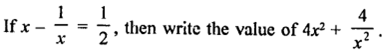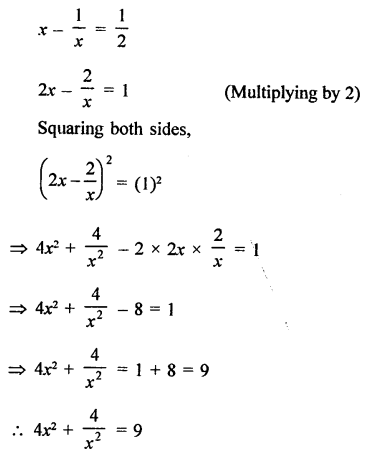Question 6 :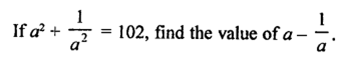Answer 6 :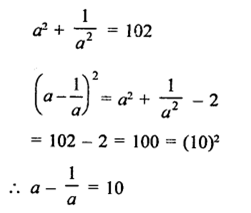Question 7 :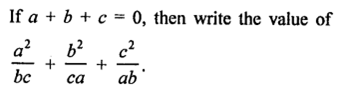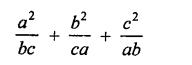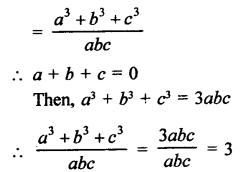Todays Deals### RD Chapter 4- Algebraic Identities Ex-VSAQS Contributorskrishan

Name:
Email:

# Latest News# 9000 interview questions in different categories